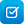# Mathematics / Year 7 / Number and Algebra / Number and place value

Curriculum content descriptions

Apply the associative, commutative and distributive laws to aid mental and written computation (ACMNA151)

Elaborations
• understanding that arithmetic laws are powerful ways of describing and simplifying calculations
General capabilities
• Numeracy Numeracy
ScOT terms

Mental arithmetic,  Distributivity,  Associativity,  Commutativity,  Written calculations

## Refine results by

Year level 7-8
Resource type
Learning area Mathematics

## Refine by topic

Related topic### Exploring order of operations

Work through mathematical operations in an algorithm. Perform calculations with integers where the order of operations is important. Start with two-step operations such as 6+10x2. Move on to numerical expressions involving several operations and notation such as parentheses and indices. Solve numerical expressions quickly ...### School canteen: two traders: level 1

Buy supplies online for a school canteen. Purchase given amounts of items such as bottles of orange juice and cheese sticks. For each item on the shopping list, check the prices and package sizes of two traders. Perform basic calculations to select the cheaper deal for each item. Compare the total cost of the items from ...### Top drawer teachers

This is a website that covers five mathematical topics: fractions, mental computation, patterns, statistical literacy and ‘reasoning’. It also explores good teaching practice linked to classroom activities including how to address common misunderstandings. There is also a section for assessment, as well as worksheets with ...### Number talks

Use these tasks and resources to provide opportunities for students to explain mental strategies for performing calculations. Present a number of mathematical statements for students to investigate whether they are true or false. As a further challenge include statements about percentages and improper fractions.### The laws of arithmetic and their use in algebra

This is a website designed for both teachers and students that refers to algebraic notation, the laws of arithmetic and the use of these laws in algebra from the Australian Curriculum for year 7 students. It contains material on algebraic notation, the commutative and associative laws, the use of brackets and the orders ...### Mental computation: ratio and percentage

This is a teacher resource that is part of a wider series; it provides a strategies-based approach to mental computation of problems involving ratios and percentages for primary level. It provides 13 classroom activities that enable students to develop mental-computation skills in sequence by building conceptual understanding ...### TIMES Module 9: Number and Algebra: multiplication of whole numbers - teacher guide

This is a 23-page guide for teachers. This module contains a description of suitable models for multiplication, a discussion of the types of problems that require multiplication for their solution, and mental and written strategies for multiplication. The use of the commutative, associative and distributive laws is described. ...### Mental computation

This is a website designed for both teachers and students that discusses methods of mental computation. In particular, applying the associative, commutative and distributive laws to aid mental and written computation is discussed. These are important ideas for the introduction of algebra. There are pages for both teachers ...### TIMES Module 7: Number and Algebra: addition of whole numbers - teacher guide

This is a 16-page guide for teachers. This module introduces addition of whole numbers.### The divider: with or without remainders

Solve divisions such as 147/7 or 157/6 (some have remainders). Use a partitioning tool to help solve randomly generated divisions. Learn strategies to do complex arithmetic in your head. Split a division into parts that are easy to work with, use times tables, then solve the original calculation.### Order of operations

This is a set of interactive questions relating to the laws of arithmetic, their usefulness in calculations, and their importance and relevance in order of operations calculations. The questions challenge and extend students' thinking about generalisations of the laws and make a link between arithmetic and algebra. The ...### Area of triangles: triangles with a right angle

Find the area of right-angled triangles. Explore how the formula works. First, estimate the area of a chosen triangle on a grid. Next, work out the correct formula by assembling a series of triangles and rectangles. Then, compare the actual area of the triangle with your original estimate. Practise applying the formula ...### Thinking division: remainders: assessment

Test your ability to turn a word problem into an equation with a remainder. Solve the equation without a calculator. For example, use a partitioning tool to help solve the equation 169÷4. Identify the remainder, which can be expressed as a whole number or a fraction. Choose which strategy you used to solve the problem. ...### The foul food maker: go figure

This tutorial is suitable for use with a screen reader. It explains how the use of simple words can describe the likelihood of everyday events. How likely is an event: certain, likely, equal chance, unlikely or certainly not? Answer some questions using these words and then build your own examples. Learn how to describe ...### TIMES Module 10: Number and Algebra: division of whole numbers - teacher guide

This is a 26-page guide for teachers. This module contains a description of suitable models for division, a discussion of the types of problems that require division for their solution, and mental and written strategies for division.### TIMES Module 19: Number and Algebra: whole number arithmetic - teacher guide

This is a 17-page guide for teachers, a module that introduces addition of whole numbers.### Brackets

A short, animated tutorial about expanding brackets, followed by an interactive quiz.### Literacy and numeracy improvement: a whole-school approach

This teacher resource describes how 74 public schools in metropolitan, regional and rural Western Australia used three major components of the school improvement cycle to achieve significant improvement in the literacy and numeracy learning outcomes of their students. The resource is organised in nine sections: Summary, ...